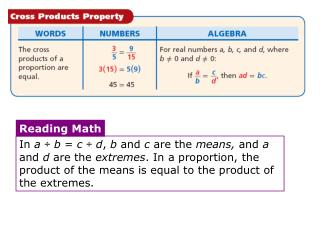DownloadDownload PresentationTélécharger la présentation- - - - - - - - - - - - - - - - - - - - - - - - - - - E N D - - - - - - - - - - - - - - - - - - - - - - - - - - -
##### Presentation Transcript

1. Reading Math In a ÷ b = c ÷ d, b and c are the means, and a and d are the extremes. In a proportion, the product of the means is equal to the product of the extremes.

2. 16 24 = 14c 1624 206.4 24p p 12.9 = = = = 88132 p12.9 2424 88c 1848 Example 1: Solving Proportions Solve each proportion. 14 c = A. B. 88132 206.4 = 24p 88c =1848 88 88 8.6 = p c = 21

3. Because percents can be expressed as ratios, you can use the proportion to solve percent problems. Remember! Percent is a ratio that means per hundred. For example: 30% = 0.30 = 30 100

4. A poll taken one day before an election showed that 22.5% of voters planned to vote for a certain candidate. If 1800 voters participated in the poll, how many indicated that they planned to vote for that candidate? Ex 2: Method 1 Use a proportion. Method 2 Use a percent equation. Percent (as decimal) whole = part 22.5(1800) = 100x 0.2251800 = x 405 = x x = 405 So 405 voters are planning to vote for that candidate.

5. 1.24 m 1 stride length 39.37 in. 1 m 49 in. 1 stride length  ≈ 600 m 482 strides x m 1 stride = A rate is a ratio that involves two different units. You are familiar with many rates, such as miles per hour (mi/h), words per minute (wpm), or dollars per gallon of gasoline. Rates can be helpful in solving many problems. Ex 3: Ryan ran 600 meters and counted 482 strides. How long is Ryan’s stride in inches? (Hint: 1 m ≈ 39.37 in.) Use a proportion to find the length of his stride in meters. 600 = 482x x ≈ 1.24 m Convert the stride length to inches. Ryan’s stride length is approximately 49 inches.

6. Z Y Reading Math B Step 1 Graph ∆XYZ. Then draw XB. The ratio of the corresponding side lengths of similar figures is often called the scale factor. X Similar figures have the same shape but not necessarily the same size. Two figures are similar if their corresponding angles are congruent and corresponding sides are proportional. ∆XYZ has vertices X(0, 0), Y(–6, 9) and Z(0, 9). Ex 4: ∆XAB is similar to∆XYZ with a vertex at B(0, 3). Graph ∆XYZ and ∆XAB on the same grid.

7. = = Z Y height of ∆XAB width of ∆XAB height of ∆XYZ width of ∆XYZ 3x A B 9 6 X Step 2To find the width of ∆XAB, use a proportion. 9x = 18, so x = 2 Step 3 To graph ∆XAB, first find the coordinate of A. The width is 2 units, and the height is 3 units, so the coordinates of A are (–2, 3).

8. = h ft 9 ft 6 ft 6 22 Shadow of tree Height of tree Shadow of house Height of house 22 ft = 9 h Example 5: Nature Application The tree in front of Luka’s house casts a 6-foot shadow at the same time as the house casts a 22-fot shadow. If the tree is 9 feet tall, how tall is the house? Sketch the situation. The triangles formed by using the shadows are similar, so Luka can use a proportion to find h the height of the house. 6h = 198 h = 33 The house is 33 feet high.